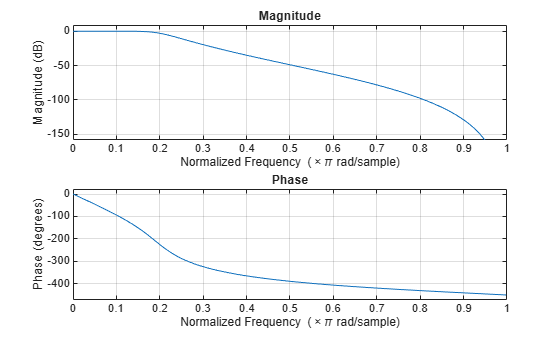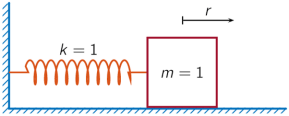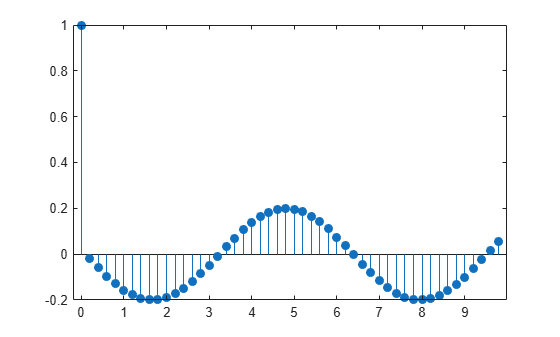Documentation

### This is machine translation

Mouseover text to see original. Click the button below to return to the English version of the page.

Note: This page has been translated by MathWorks. Click here to see
To view all translated materials including this page, select Country from the country navigator on the bottom of this page.

# ss2sos

Convert digital filter state-space parameters to second-order sections form

## Syntax

```[sos,g] = ss2sos(A,B,C,D) [sos,g] = ss2sos(A,B,C,D,iu) [sos,g] = ss2sos(A,B,C,D,'order') [sos,g] = ss2sos(A,B,C,D,iu,'order') [sos,g] = ss2sos(A,B,C,D,iu,'order','scale') sos = ss2sos(...) ```

## Description

`ss2sos` converts a state-space representation of a given digital filter to an equivalent second-order section representation.

`[sos,g] = ss2sos(A,B,C,D)` finds a matrix `sos` in second-order section form with gain `g` that is equivalent to the state-space system represented by input arguments `A`, `B`, `C`, and `D`.

### Note

The input state-space system must be single-output and real.

`sos` is an L-by-6 matrix

`$\text{sos}=\left[\begin{array}{cccccc}{b}_{01}& {b}_{11}& {b}_{21}& 1& {a}_{11}& {a}_{21}\\ {b}_{02}& {b}_{12}& {b}_{22}& 1& {a}_{12}& {a}_{22}\\ ⋮& ⋮& ⋮& ⋮& ⋮& ⋮\\ {b}_{0L}& {b}_{1L}& {b}_{2L}& 1& {a}_{1L}& {a}_{2L}\end{array}\right]$`

whose rows contain the numerator and denominator coefficients bik and aik of the second-order sections of H(z).

`$H\left(z\right)=g\prod _{k=1}^{L}{H}_{k}\left(z\right)=g\prod _{k=1}^{L}\frac{{b}_{0k}+{b}_{1k}{z}^{-1}+{b}_{2k}{z}^{-2}}{1+{a}_{1k}{z}^{-1}+{a}_{2k}{z}^{-2}}$`

`[sos,g] = ss2sos(A,B,C,D,iu)` specifies a scalar `iu` that determines which input of the state-space system `A`, `B`, `C`, `D` is used in the conversion. The default for `iu` is 1.

`[sos,g] = ss2sos(A,B,C,D,'order')` and

`[sos,g] = ss2sos(A,B,C,D,iu,'order')` specify the order of the rows in `sos`, where `'order'` is

• `'down'`, to order the sections so the first row of `sos` contains the poles closest to the unit circle

• `'up'`, to order the sections so the first row of `sos` contains the poles farthest from the unit circle (default)

The zeros are always paired with the poles closest to them.

`[sos,g] = ss2sos(A,B,C,D,iu,'order','scale')` specifies the desired scaling of the gain and the numerator coefficients of all second-order sections, where `'scale'` is

• `'none'`, to apply no scaling (default)

• `'inf'`, to apply infinity-norm scaling

• `'two'`, to apply 2-norm scaling

Using infinity-norm scaling in conjunction with `up`-ordering minimizes the probability of overflow in the realization. Using 2-norm scaling in conjunction with `down`-ordering minimizes the peak round-off noise.

### Note

Infinity-norm and 2-norm scaling are appropriate only for direct-form II implementations.

`sos = ss2sos(...)` embeds the overall system gain, `g`, in the first section, H1(z), so that

`$H\left(z\right)=\prod _{k=1}^{L}{H}_{k}\left(z\right)$`

### Note

Embedding the gain in the first section when scaling a direct-form II structure is not recommended and may result in erratic scaling. To avoid embedding the gain, use `ss2sos` with two outputs.

## Examples

collapse all

Design a 5th-order Butterworth lowpass filter using the `butter` function. Specify a cutoff frequency of $0.2\pi$ rad/sample. Express the output in state-space form. Convert the state-space result to second-order sections. Visualize the frequency response of the filter.

```[A,B,C,D] = butter(5,0.2); sos = ss2sos(A,B,C,D)```
```sos = 3×6 0.0013 0.0013 0 1.0000 -0.5095 0 1.0000 2.0012 1.0012 1.0000 -1.0966 0.3554 1.0000 1.9968 0.9968 1.0000 -1.3693 0.6926 ```
`freqz(sos)`A one-dimensional discrete-time oscillating system consists of a unit mass, $m$, attached to a wall by a spring of unit elastic constant. A sensor measures the acceleration, $a$, of the mass.The system is sampled at ${F}_{s}=5$ Hz. Generate 50 time samples. Define the sampling interval $\Delta t=1/{F}_{s}$.

```Fs = 5; dt = 1/Fs; N = 50; t = dt*(0:N-1);```

The oscillator can be described by the state-space equations

`$\begin{array}{c}x\left(k+1\right)=Ax\left(k\right)+Bu\left(k\right),\\ y\left(k\right)=Cx\left(k\right)+Du\left(k\right),\end{array}$`

where $x={\left(\begin{array}{cc}r& v\end{array}\right)}^{T}$ is the state vector, $r$ and $v$ are respectively the position and velocity of the mass, and the matrices

`$A=\left(\begin{array}{cc}\mathrm{cos}\Delta t& \mathrm{sin}\Delta t\\ -\mathrm{sin}\Delta t& \mathrm{cos}\Delta t\end{array}\right),\phantom{\rule{1em}{0ex}}B=\left(\begin{array}{c}1-\mathrm{cos}\Delta t\\ \mathrm{sin}\Delta t\end{array}\right),\phantom{\rule{1em}{0ex}}C=\left(\begin{array}{cc}-1& 0\end{array}\right),\phantom{\rule{1em}{0ex}}D=\left(\begin{array}{c}1\end{array}\right).$`

```A = [cos(dt) sin(dt);-sin(dt) cos(dt)]; B = [1-cos(dt);sin(dt)]; C = [-1 0]; D = 1;```

The system is excited with a unit impulse in the positive direction. Use the state-space model to compute the time evolution of the system starting from an all-zero initial state.

```u = [1 zeros(1,N-1)]; x = [0;0]; for k = 1:N y(k) = C*x + D*u(k); x = A*x + B*u(k); end```

Plot the acceleration of the mass as a function of time.

`stem(t,y,'filled')`Compute the time-dependent acceleration using the transfer function to filter the input. Express the transfer function as second-order sections. Plot the result.

```sos = ss2sos(A,B,C,D); yt = sosfilt(sos,u); stem(t,yt,'filled')```The result is the same in both cases.

## Algorithms

`ss2sos` uses a four-step algorithm to determine the second-order section representation for an input state-space system:

1. It finds the poles and zeros of the system given by `A`, `B`, `C`, and `D`.

2. It uses the function `zp2sos`, which first groups the zeros and poles into complex conjugate pairs using the `cplxpair` function. `zp2sos` then forms the second-order sections by matching the pole and zero pairs according to the following rules:

1. Match the poles closest to the unit circle with the zeros closest to those poles.

2. Match the poles next closest to the unit circle with the zeros closest to those poles.

3. Continue until all of the poles and zeros are matched.

`ss2sos` groups real poles into sections with the real poles closest to them in absolute value. The same rule holds for real zeros.

3. It orders the sections according to the proximity of the pole pairs to the unit circle. `ss2sos` normally orders the sections with poles closest to the unit circle last in the cascade. You can tell `ss2sos` to order the sections in the reverse order by specifying the `'down'` flag.

4. `ss2sos` scales the sections by the norm specified in the `'``scale``'` argument. For arbitrary H(ω), the scaling is defined by

`${‖H‖}_{p}={\left[\frac{1}{2\pi }\underset{0}{\overset{2\pi }{\int }}{|H\left(\omega \right)|}^{p}d\omega \right]}^{1/p}$`

where p can be either ∞ or 2. See the references for details. This scaling is an attempt to minimize overflow or peak round-off noise in fixed point filter implementations.

## References

 Jackson, L. B. Digital Filters and Signal Processing. 3rd Ed. Boston: Kluwer Academic Publishers, 1996, chap. 11.

 Mitra, S. K. Digital Signal Processing: A Computer-Based Approach. New York: McGraw-Hill, 1998, chap. 9.

 Vaidyanathan, P. P. “Robust Digital Filter Structures.” Handbook for Digital Signal Processing (S. K. Mitra and J. F. Kaiser, eds.). New York: John Wiley & Sons, 1993, chap. 7.